DAT Perceptual Ability : View Recognition / Top-Front-End Questions

Example Questions

Example Question #1 : View Recognition / Top Front End Questions

The pictures that follow are top, front, and end views of solid objects. The views are without perspective. That is, the points in the viewed surface are viewed along parallel lines of vision. The projection looking DOWN on it is shown in the upper left-hand corner (TOP VIEW). The projection looking at the object from the FRONT is shown in the lower left-hand corner (FRONT VIEW). The projection looking at the object from the END is shown in the lower right-hand corner. (END VIEW). These views are ALWAYS in the same positions and are labeled accordingly.

Note that lines that cannot be seen on the surface in some particular view are DOTTED in that view.

In these problems, two views are shown, with four alternatives to complete the set. You are to select the correct one. It is not always the end view that must be selected; sometimes it is the top view or the front view that is missing.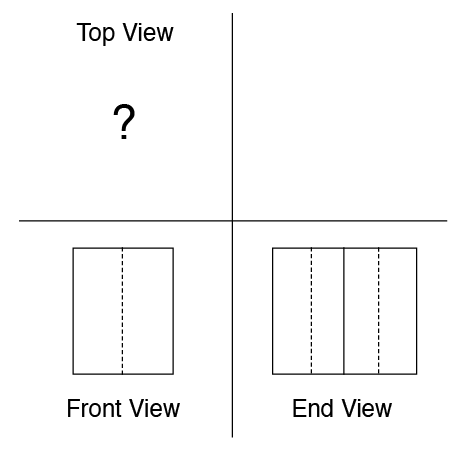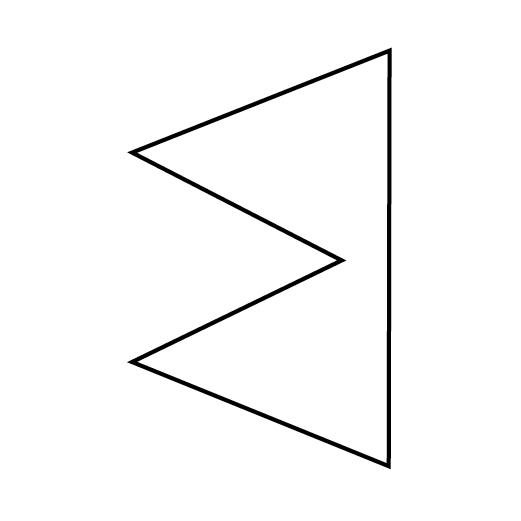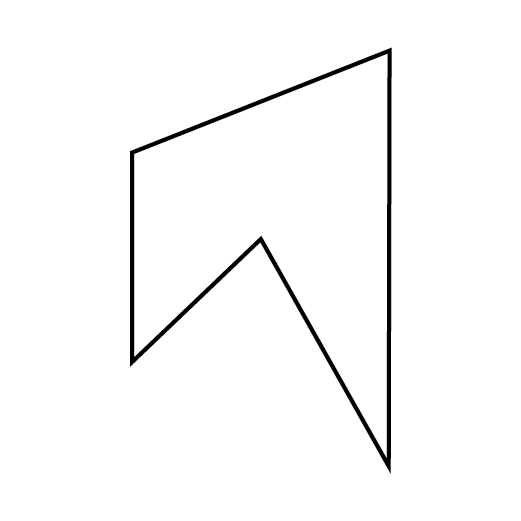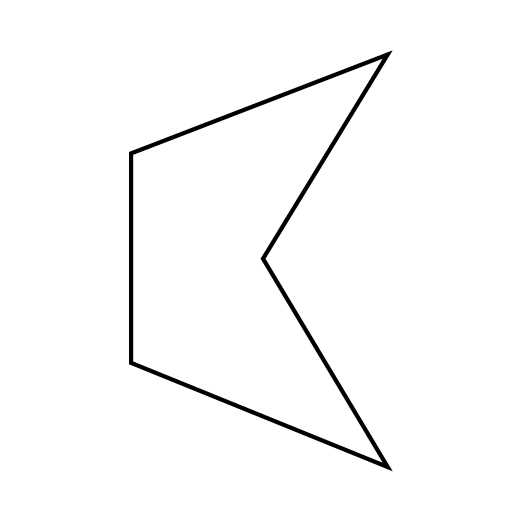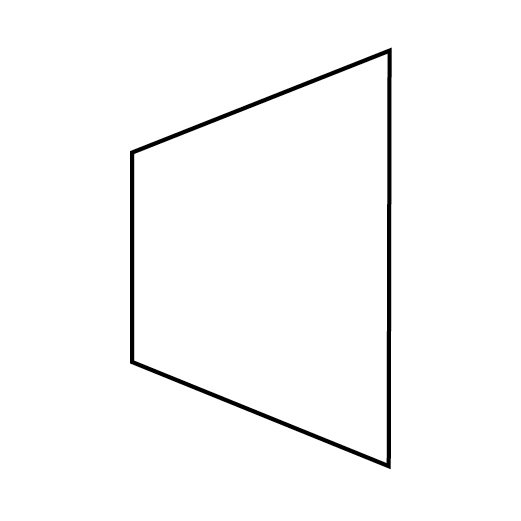Explanation:

To answer this question correctly, you need to understand the difference between solid lines and dashed lines in View Recognition/Top-Front-End questions. Solid lines refer to lines and edges that you can see in a particular view, whereas dashed lines refer to lines and edges that are obscured by something in front of them from that particular view. One tricky part about this question is that when shapes involve angles, the edge on which the vertex of the angle is located is also represented by a line, solid or dotted as necessary.

Considering the front view, we're shown a rectangular face bisected by a dotted line. This tells us that an angle is involved. If a shape built from ninety-degree angles were involved, an even number of lines would be necessary. Since we only see one line, we know that two faces must be coming to a point, either pointing outward from the face we're looking at directly, or inward. It's impossible to tell from just this view. Furthermore, the shape could be an equilateral triangle if these angled edges started at the corners we can see, but alternatively, those edges could extend in space into a prism for a while before angling toward that point. Again, it's impossible to tell from this view alone since these extensions and differences would all occur exactly on the edges we're looking at directly, and thus they wouldn't be shown in this diagram.

Let's now consider what we learn when we combine what we know from the Front View with what we learn from the End View. Now there are three vertical lines. One of them, the center one, is solid, so we are looking head-on at two angled faces. The point could either be coming toward us, away from the shape, or it could extend into the shape. We can't tell. The dotted lines tell us that there are two edges behind the face we're looking at. We don't know if these edges are in line with each other or not, and if they're not, we can't tell which one is closer to us.

Let's look at the answer choices. Since there are so many options as to what these diagrams could represent, let's use process of elimination to figure out which answer choices can't be correct and which one must be correct.This shape can't be correct. While the face at bottom lines up with what we see from the end view (two angled faces meeting in the middle of the shape), the far side of the shape only has one face, not three. We need to see three different faces on the far side of this shape because there are two vertical dotted lines shown in the End View diagram.This shape can't be correct either. While it would involve three faces behind the immediate view if we looked at the right side head-on, there is no angle like the one represented in the Front View diagram. Plus, this diagram is pointed in the wrong direction! Direction is important in Front-End-Side problems.This shape is also incorrect. If we looked at the shape from the bottom, the triangular "indent" would extend much farther to the right of center than is shown in the Front View diagram. In addition, if we looked head-on at the right face of the diagram, we wouldn't see any edges that would match up with the solid vertical line in the End View diagram. That line would have to be dashed like the other two for the End View diagram to be correct.This is the correct answer. If we look at the shape from the front, we see that the triangular indent extends to halfway between the solid vertical line that forms the left face and the points of the rightmost part of the shape. The vertical line would be represented by the left vertical line in the Front View, and the right vertical line would be represented by a line extending downward from the "points" of the right side of the shape. Since these points are in line with one another, only one line would be required for the right side in the Front View diagram. Seen from the right for the End View, the solid vertical line in the middle represents the middle of the indented angle, and the two vertical lines represent the edges where on the left part of the top view, the sides angle out from the vertical line.

Example Question #2 : View Recognition / Top Front End Questions

The pictures that follow are top, front, and end views of solid objects. The views are without perspective. That is, the points in the viewed surface are viewed along parallel lines of vision. The projection looking DOWN on it is shown in the upper left-hand corner (TOP VIEW). The projection looking at the object from the FRONT is shown in the lower left-hand corner (FRONT VIEW). The projection looking at the object from the END is shown in the lower right-hand corner. (END VIEW). These views are ALWAYS in the same positions and are labeled accordingly.

Note that lines that cannot be seen on the surface in some particular view are DOTTED in that view.

In these problems, two views are shown, with four alternatives to complete the set. You are to select the correct one. It is not always the end view that must be selected; sometimes it is the top view or the front view that is missing.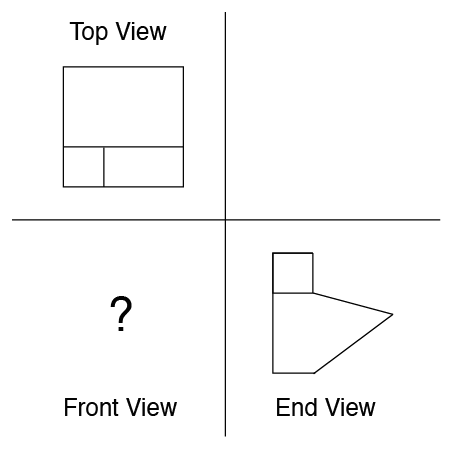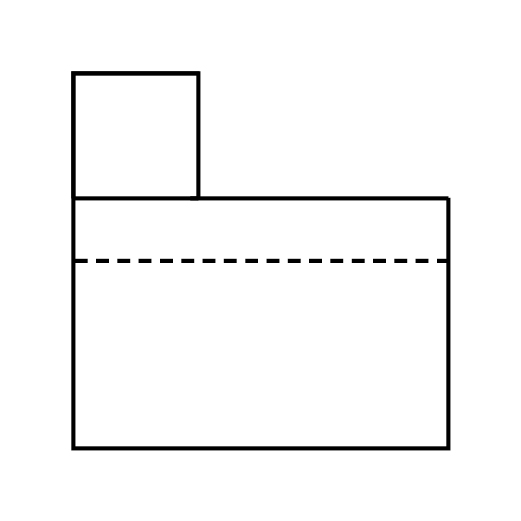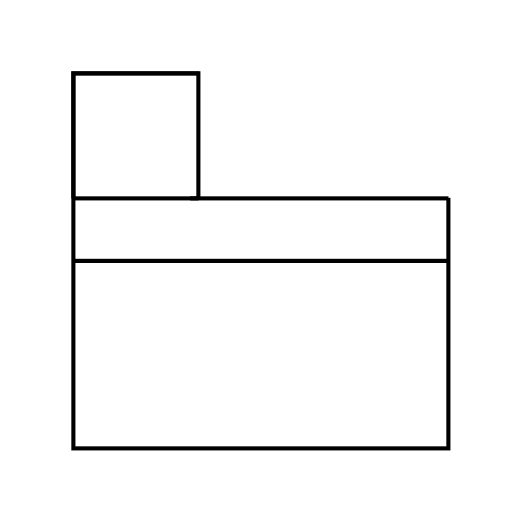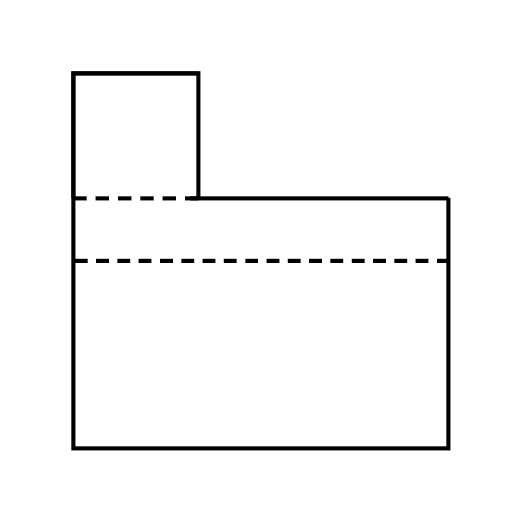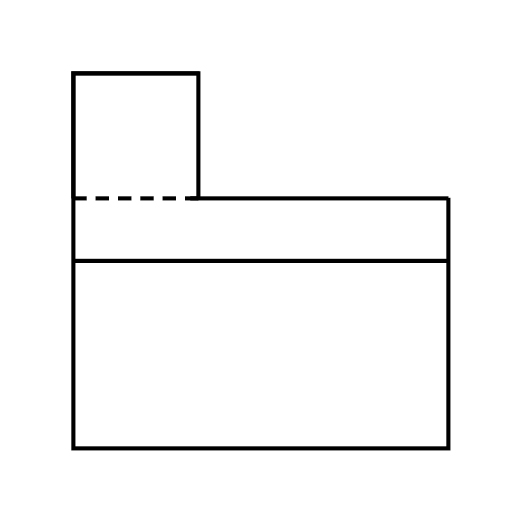Explanation:

To answer this question correctly, you need to understand the difference between solid lines and dashed lines on the DAT Perceptual Ability section. In this case, we're looking for the front view. The top view of this shape shows us that it is divided into three sections. Considering just this view, this could mean a variety of different things: the shape might be cube-like and each section might be a different step, for example, but we'd have no information about how the heights of each "step" would compare. In addition, the shape might not be a cube; angles might be involved. We can't tell solely from looking at the top view, though. The end view provides us with more information that allows us to narrow down our interpretation of the top view. Here, we see that the shape, when viewed from the end, involves an angle, and that there is a solid line at the bottom of the cube above the angular piece. This tells us that the cube is not connected to the shape that includes the angle. The cube's face isn't connected to the end face of the angled piece; it is separate. This tells us that the cube is farther away than the angled end face facing us directly in the end view. If one of its faces were connected to the angled end face, the diagram would not include a line separating the "cube" part of the shape from the angled part of the shape.

Now let's consider what the front of the shape has to look like. Notice that the "cube" part of the shape is on the left in all of the answer choices. This is important, as it tells us that we are looking at the flat, rectangular side of the larger part of the shape head-on, not at the angled sides. If we were looking at the angled sides, the "cube" part would have to be on the right side of the front-view diagram, and this isn't an option in any of the answer choices.

So, we know that the line forming the vertex of the angle shown in the End View diagram is behind the face of the shape we're directly looking at in the Front View. This tells us that this line must be dotted, not solid. Figuring this out allows us to ignore the following two answer choices because they use solid lines for their bottom-most shape-crossing line:This leaves us with two remaining answer choices, each of which correctly uses a dotted line:The only difference between these two answer choices is that the one on the left uses a dashed line to divide the "cube" shape from the rest of the shape, and the one on the right uses a solid line for this division. Which should it be? Consider how this "cube" shape will appear when we look at the shape head-on with the face shown as the bottom line of the Top View appearing first. Is the "cube" part of the shape in line with this face, or is it located further back in space? If the former is true, we need to use a dotter line to show that the cube shape does not continue for the entire volume of the shape, but that there is negative space located behind it. If the latter is true, we need to use a solid line to show that the negative space is in front of the cube. Looking at the Top View diagram, we can see that one face of the cube will be even with the face of the larger part of the shape at which we are looking in the Front View. This means that the negative space appears behind the cube, not in front of it, making the correct answer the shape that uses the dotted line: